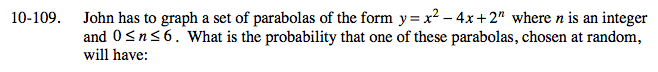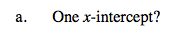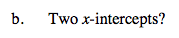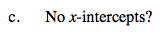### Home > A2C > Chapter Ch10 > Lesson 10.2.4 > Problem10-109

10-109.For the following, test the possible equations on your calculator or on graph paper.$P\text{(one)=\frac{1}{7}}$

What is the value of n?$P\text{(two)=\frac{2}{7}}$

What are the values of n?$P\text{(none)=\frac{4}{7}}$

What are the values of n?